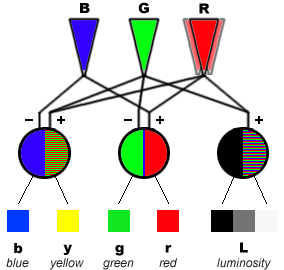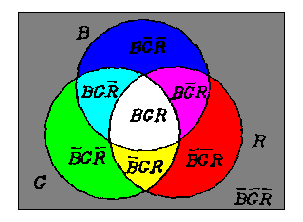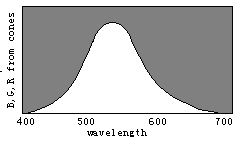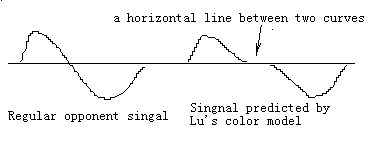Problems and Way out of Zone ModelsThe right picture is the reversed one of the left picture. This shows that real opponet colors are Red/Cyan and Green/Magenta instead of Red/Green.from http://webvision.med.utah.edu/KallColor.htmlFrom http://www.handprint.com/HP/WCL/color1.html,Thanks. Zone Model I:  B,G,R result in White/Black The problem: G, R cannot be opponent or cannot compose white as indicated by Herring (B,G,R)=(0,0,1) should be typical red color, there should be only output r>0. But The above model also yields y>0. Zone Model II: G, R result White/Black, and Red/Green( actully green here is Cyan; or R is magenta) Problem: White can be produced without B or S signal--not compatible with Young-Helmholtz's theory and Color optics.  The output r should be magenta otherwise r and g are not opponent.(B,G,R)=(1,0,0) should be typical blue color. There should be only output b>0. But The above model also yields r>0. How to get Unique Colors? See decomposition of a color: (B,G,R)=c1*(0,0,1)+c2*(0,1,1)+....c7(1,1,1) for example: (3,5,9)=3*(1,1,1)+2*(0,1,1)+4*(0,0,1)=3*white+2*yellow+4*red Problem is how to get ci, i=1,2,...,7, which cannot be obtained only by additions and subtractions. The way out is: using logical operations to replace arithmetic opertionsVen's Diagram to show binary logical operations: M=RGVRBVBG R-M=RED/Cyan;G-N=Green/Magenta; B-M=Blue/Yellow3-8 decoding model: Continously logical operations: M=middle(R,G,B)=max(min(R,G),min(R,B),min(R,B)) R-M=RED/Cyan;G-M=Green/Magenta; B-M=Blue/Yellow New problem is: there are three pairs of opponent colors. My explanation: michanism of color vision is evolving. Green/Magenta and Red/Cyan are not apart to much. So when we test opponent signals, we always confuse them each other.Decoding model can unify Young-Helmholtz's theory and Herring's  Theory better, and also explain evolution of color vision better Opponent color signals change wavelength Critcal test: The decoding model predicts both above opponent signals' existence. The left one exists because color vision is evolving, some low level processes remain.

Chenguang Lu survival@163.net